# Pandas Difference Between two Dataframes

There are often cases where we need to find out the common rows between the two dataframes or find the rows which are in one dataframe and missing from second dataframe. In this post we will see how using pandas we can achieve this.

However if you are interested to compare the column between two dataframes then check out this post.

Here are two dataframes which we will use to find common rows, Rows in dataframe 1 and Rows in dataframe 2.

``````import pandas as pd

df1 = pd.DataFrame({'City': ['New York', 'Chicago', 'Tokyo', 'Paris','New Delhi'],
'Temp': [59, 29, 73, 56,48]})

df2 = pd.DataFrame({'City': ['London', 'New York', 'Tokyo', 'New Delhi','Paris'],
'Temp': [55, 55, 73, 85,56]})
``````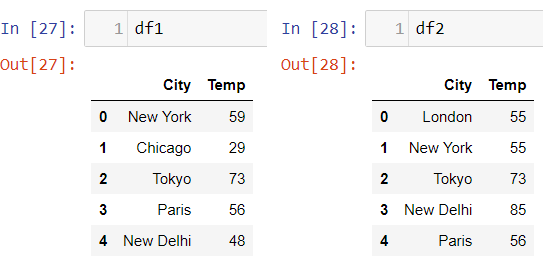## Find Common Rows between two Dataframe Using Merge Function

Using the merge function you can get the matching rows between the two dataframes. So we are merging dataframe(df1) with dataframe(df2) and Type of merge to be performed is inner, which use intersection of keys from both frames, similar to a SQL inner join.

``````df = df1.merge(df2, how = 'inner' ,indicator=False)
df
``````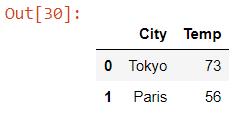So what we get is Tokyo and Paris which is common between the two dataframes

## Find Common Rows Between Two Dataframes Using Concat Function

The concat function concatenate second dataframe(df2) below the first dataframe(df1) along a particular axis with optional set logic along the other axes. So here we are concating the two dataframes and then grouping on all the columns and find rows which have count greater than 1 because those are the rows common to both the dataframes. here is code snippet:

``````df = pd.concat([df1, df2])

df = df.reset_index(drop=True)

df_gpby = df.groupby(list(df.columns))

idx = [x for x in df_gpby.groups.values() if len(x) != 1]
df.reindex(idx)
``````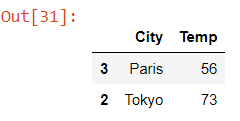## Find Rows in DF1 Which Are Not Available in DF2

We will see how to get all the rows in dataframe(df1) which are not available in dataframe(df2). We can use the same merge function as used above only the parameter indicator is set to true, which adds a column to output DataFrame called “_merge” with information on the source of each row. If string, column with information on source of each row will be added to output DataFrame, and column will be named value of string. Information column is Categorical-type and takes on a value of “left_only” for observations whose merge key only appears in ‘left’ DataFrame, “right_only” for observations whose merge key only appears in ‘right’ DataFrame, and “both” if the observation’s merge key is found in both

``````df = df1.merge(df2, how = 'outer' ,indicator=True).loc[lambda x : x['_merge']=='left_only']

df
``````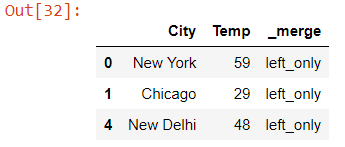Using the lambda function we have filtered the rows with _merge value “left_only” to get all the rows in df1 which are missing from df2

## Find Rows in DF2 Which Are Not Available in DF1

Just change the filter value on _merge column to right_only to get all the rows which are available in dataframe(df2) only and missing from df1

Just see the type of merge i.e. parameter how is changed to outer which is basically union of keys from both frames, similar to a SQL full outer join

``````df = df1.merge(df2, how = 'outer' ,indicator=True).loc[lambda x : x['_merge']=='right_only']

df
``````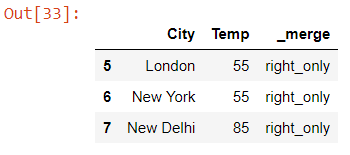## Check If Two Dataframes Are Exactly Same

In order to check if two dataframes are equal we can use equals function, which llows two Series or DataFrames to be compared against each other to see if they have the same shape and elements. NaNs in the same location are considered equal. The column headers do not need to have the same type, but the elements within the columns must be the same dtype

``````df2.equals(df1)

False
``````

## Check If Columns of Two Dataframes Are Exactly Same

Using equals you can also compare if the columns of two dataframes are equal or not

``````df2['Temp'].equals(df1['Temp'])

False
``````

## Find Rows Which Are Not common Between Two dataframes

So far we have seen all the ways to find common rows between two dataframes or rows available in one and missing from another dataframe. Now if we have to get all the rows which are not common between the two dataframe or we want to see all the unique un-matched rows between two dataframe then we can use the concat function with drop_duplicate.

``````pd.concat([df1,df2]).drop_duplicates(keep=False)
``````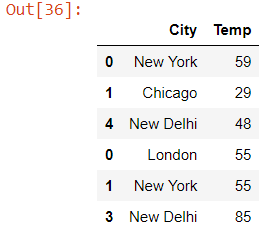## Find All Values in a Column Between Two DataframesWhich Are Not Common

We will see how to get the set of values between columns of two dataframes which aren’t common between them. So here we are finding the symmetric difference also known as the disjunctive union, of two sets is the set of elements which are in either of the sets and not in their intersection

``````set(df1.Temp).symmetric_difference(df2.Temp)

{29, 48, 55, 59, 85}
``````

The above line of code gives the not common temperature values between two dataframe and same column. Check df1 and df2 and see if the uncommon values are same.

## Conclusion

So we have seen using Pandas - Merge, Concat and Equals how we can easily find the difference between two excel, csv’s stored in dataframes. Also it gives an intuitive way to compare the dataframes and find the rows which are common or uncommon between two dataframes. You can also read this post and see how two exel files can be compared using pandas cell by cell and store the results in an excel report.

Tags:

Categories:

Updated: